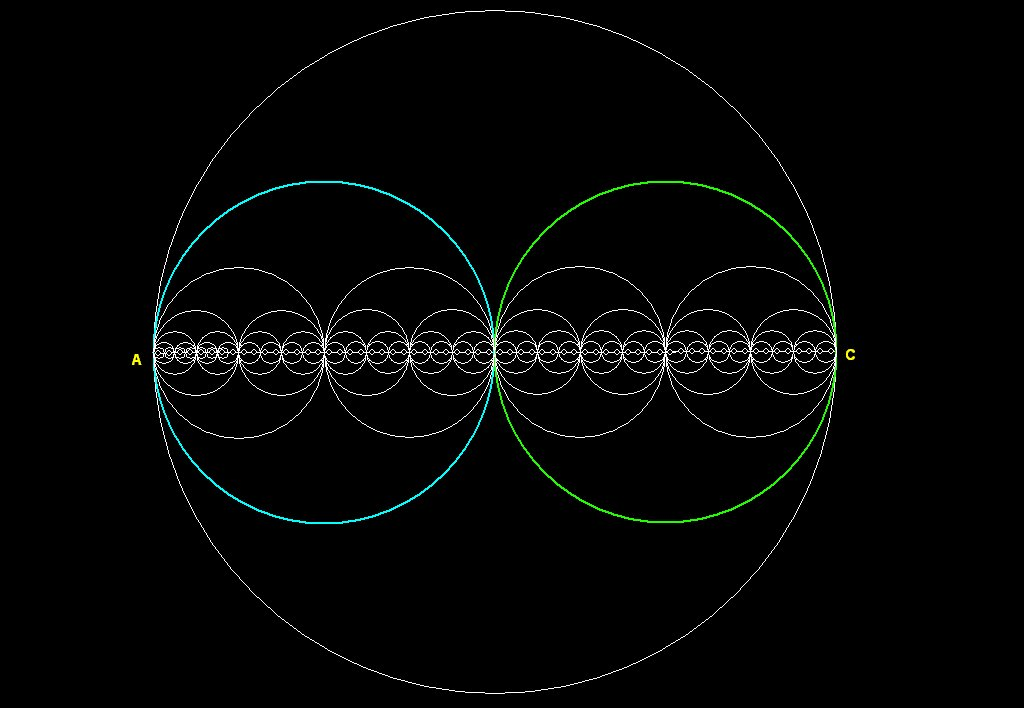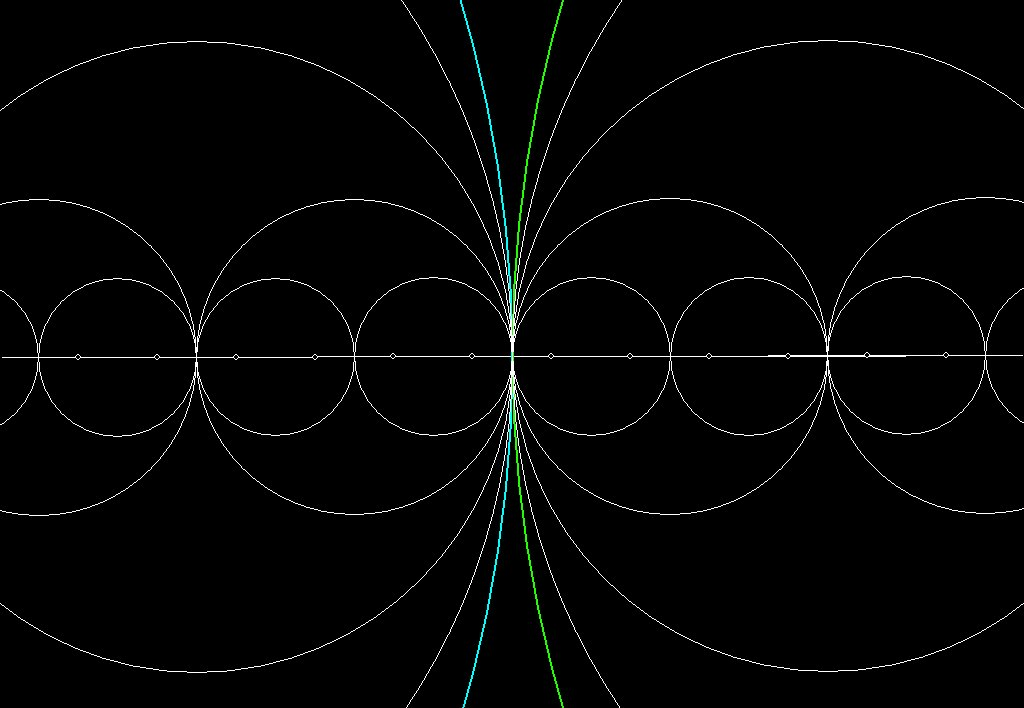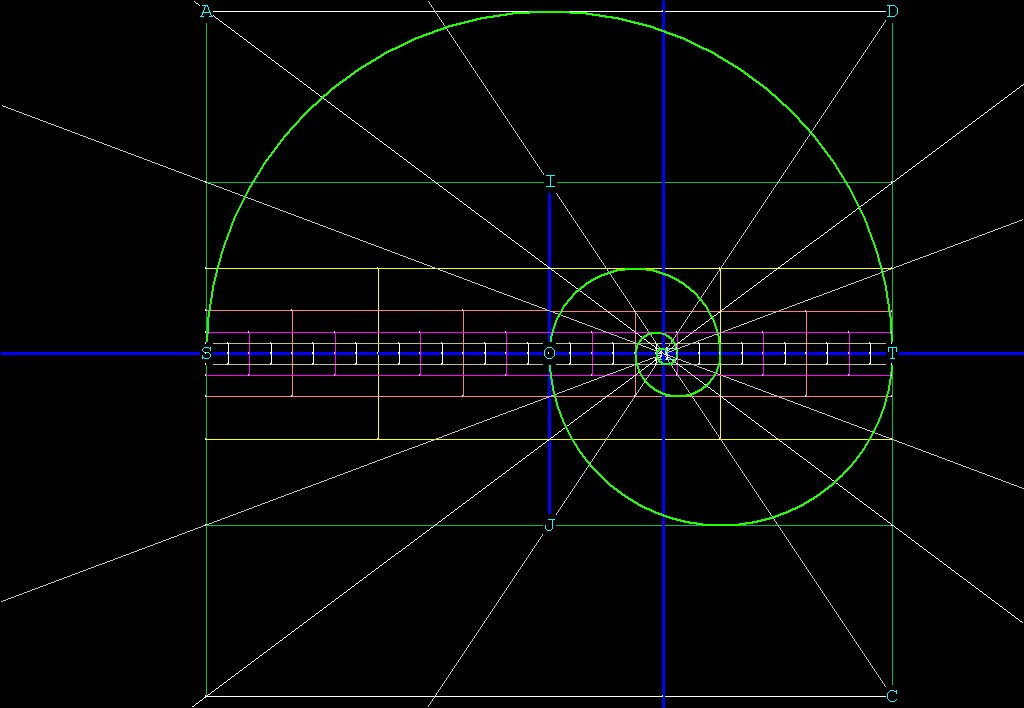The Binary Circle/Sphere PatternFigure 1 -- The Binary circle/sphere Pattern
The binary circle/sphere pattern is composed of 2 circles inside a larger circle, such that the two circles are exactly tangent to themselves and the larger circle.

Scale

In the above diagram we have a basic pattern: a circle into which 2 smaller circles of exactly r/2 are inserted. This pattern is repeated as many times as my geometry program would allow. As the pattern is iterated, the circles cluster around the line AC and become vanishingly small.
The pattern is self-similar, meaning that it looks the same no matter how many times it is magnified, but it is not a fractal pattern. Its dimension is always 2.

This pattern has some interesting characteristics, and it got me thinking about consciousness, scales of magnitude and observation.
If you start from A and go up on the blue circle, clockwise, 180 degrees and come to the middle, then go down on the green circle and come to C, then from C go around the green circle counter-clockwise 180 back to the middle, then down the bottom half of the blue circle back to A, you will have made a complete circuit of both circles. The path traveled is exactly equal to the circumference of the big circle. This can be seen by noting that if AC = 2, then r = 1 for the big circle, the circumference of the big circle  is 2*Pi. The circumference of the blue and green circles is Pi,   (2* Pi * r/2) and so the total distance traveled around the 2 circles is 2Pi.
We can continue this pattern of traversing the circumference of the smaller circles indefinitely, and the total distance will always equal 2Pi.
The total number of circles upon each magnification will be 2^n, and the circumference around each one will  be (2 * Pi)  / 2^n.
For the total circumference around all of the circles, we can write
Tc = 2^n * (Pi * 2 / 2^n)  = 2Pi, no matter how many times we magnify.

Even though this is unremarkable mathematically, it IS remarkable geometrically, because as the circles grow smaller and smaller, the path along the circumference of the circles flattens out and appears to our eyes, after only 10 iterations, to be a straight line. So the total distance around the circumference appears to be from A to C in a straight line, and back from C to A again in a straight line. To our eyes at 'real world' magnification, it then appears that Circumference = 2*D.
By reducing the scale, we have introduced inaccuracies into our observation of reality.

Of course, we have microscopes and telescopes to help us resolve these questions of scale. However, even with scanning tunneling microscopes, we can observe individual atoms and even manipulate them, but we cannot yet see clearly inside an atom, so we don’t really know for sure what’s happening at the subatomic level. It’s on a scale level that is too far removed from us.  Similarly for our observation of galaxies, and the universe at large. This diagram serves to illustrate that the only things we can really be sure of in the physical universe are things we can perceive directly.

Characteristics of the binary circle/sphere pattern

0) The pattern is self-similar; that is, no matter which point it is viewed from, or how deep is the magnification, it looks the same. The pattern is not a fractal, because it's dimension is always 2. (See Maths Section). The number of circles doubles after each iteration.

1) The pattern is an oscillator. As the spiral travels inward (see Figure 2), r, the distance from the center of the spiral to any point on it, oscillates around a point, but never reaches it.
1A) The pattern also shows curious left-and right-handed 'spirals' which always touch the same point every 360 degrees of revolution.

2) The pattern is a geometric representation of a simple binary search algorithm, eliminating upon each iteration exactly half of the remaining points on the line AC.

3) Notice the collapsing and expanding oscillating wave fronts: start at A or C. The increasing or decreasing circle  radii denote an expanding or collapsing wave front which describe a number of behaviors:
A) Wavefronts reach  greatest expansion in the middle, then collapse to A or C on the other side.
B) Wavefronts can expand all the way to A or C, then begin the same expansion process in the opposite direction.
C) Wavefronts may expand or contract at any point to any point, then expand or contract back in the opposite direction.

4) Decreasing circle/sphere radii sets up waveforms which reach higher and higher frequencies with smaller and smaller amplitudes, as the circles get smaller and smaller and more packed together. Even though the line AC appears perfectly straight, a particle traveling along it would have to wiggle back and forth. The diagram illustrates that a perfectly straight line cannot exist in nature, for it has no persistence. In other words, travel along a perfectly straight line would be instantaneous. It is, in effect, a wormhole or portal which allows perfect communication.
Anything that has persistence must have change of direction, which generates time. If this is true, then in order to exist at all, a Form must have curvature.

5) Waveforms are  'paired'  each with an opposite waveform.
Observe the blue and green circles. Start at A and go up the blue circle and down the green circle. Then equal and opposite waveform goes back up the green circle and down the blue circle, exactly balancing (and zero-ing, if the two waves are added together) the original waveform.
Shows that every energy has its opposite, to balance it out.

6) The pattern shows what looks like magnetic lines of force orthogonal to the wavefronts.
(See Figure 1A just below). Note that there is really no differentiation between the 2 forces : they ARE the pattern.Figure 1A -- Electromagnetic energy? The waveforms from left to right might represent electrical energy, the magnetic lines of force orthogonal to them represent magnetic force, but eventually curl around and become part of the pattern. Each point on the pattern generates lines of force which eventually come back to the pattern.
So the pattern is closed, but self-generating.

Below, in Figure 2, is what a binary spiral looks like:Figure 2 -- A Binary Spiral from the pattern in Figure 1. All of the circles/spheres      from Figure 1 fit inside the squares.

The binary spiral is generated by making squares around all of the circles in the pattern. Wherever you see a circle in Figure 1, just make a square around that circle and you will have Figure 2.

On to  Maths Section  for a mathematical description of the spiral.